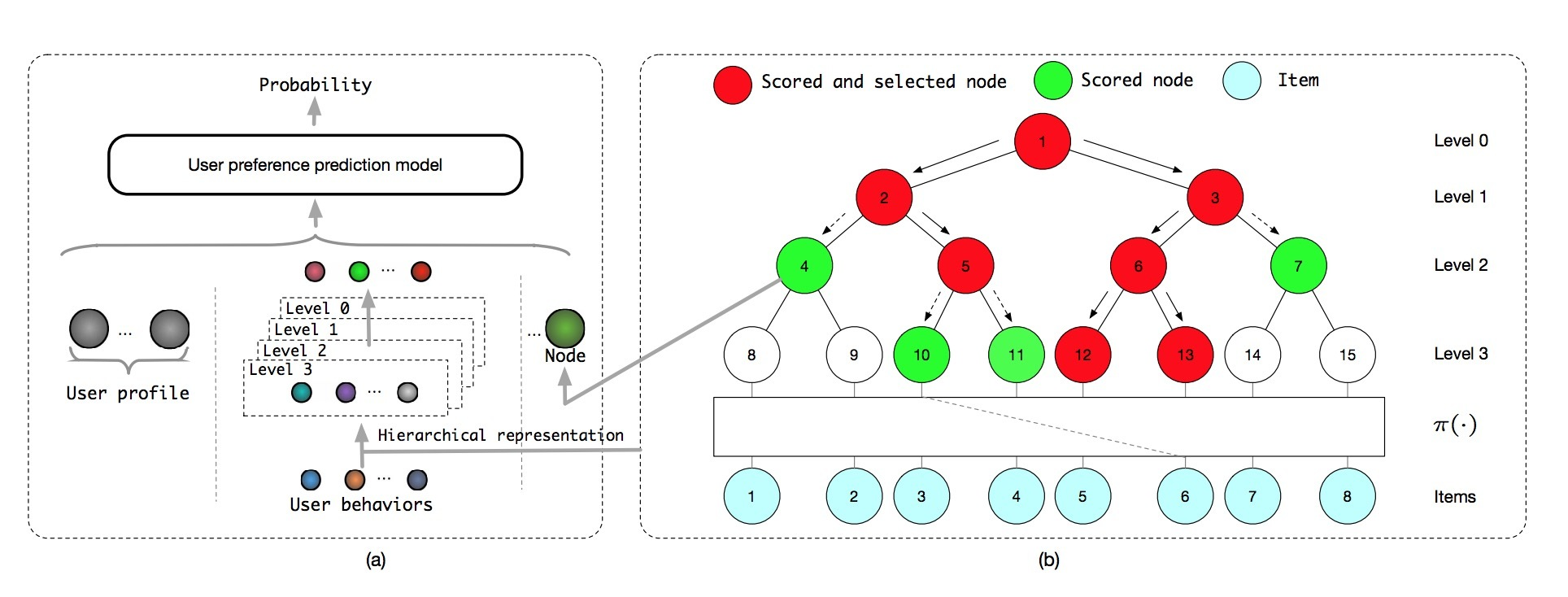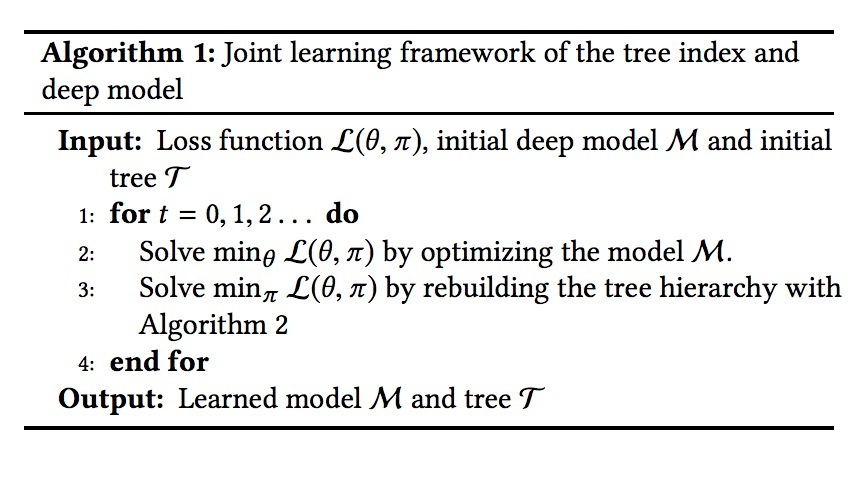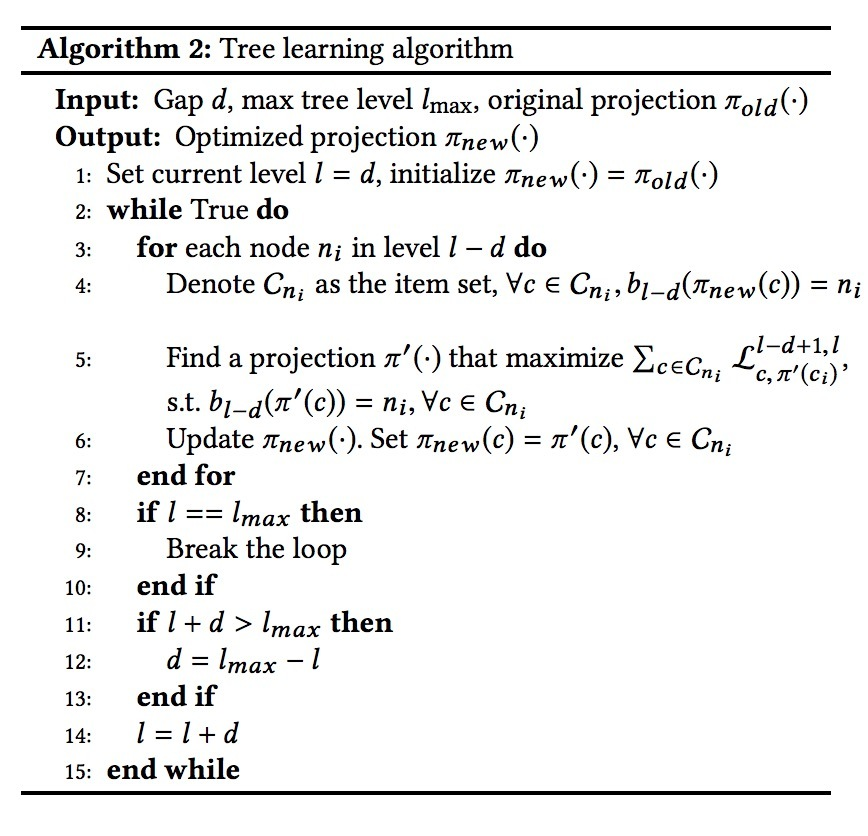介绍

index结构在kNN-based搜索、tree-based方法中扮演着不同的角色。在kNN搜索中，user和item的向量表示会首先通过学习得到，接着建立vector search index。而在tree-based方法中，tree-index的结构(hierarchy)也会影响检索模型的训练。因此，如果对tree index和用户偏好模型进行联合训练是一个重要的问题。tree-based方法在学术上也是一个活跃的话题。在已经存在的tree-based方法中，会学到tree结构，以便在在样本/标签空间（sample/label space）中得到一个更好的结构(hierarchy)。然而，在tree-learning阶段，sample/label划分任务的目标与最终目标（比如：精准推荐）并不完全一致。index learning与prediction模型训练间的不一致，会导致整个系统达到一个次优的状态。为了解决该挑战，更好地将tree index和用户偏好预测相协调，我们的工作聚焦于：通过对一个统一的偏好measure进行最优化，来开发一种同时学习树层级结构（tree hierearchy）和用户偏好预测模型。该paper的主要贡献可以归纳为：

• 我们提出了一种joint optimization框架，为tree-based推荐学习树结构和用户偏好预测模型，其中，会对一个统一的performance measure(比如：用户偏好的accuracy)进行最优化
• 我们演示了提出的树结构学习算法，它等同于二分图(bipartite graph)的最大权匹配（max weighted matching）问题，并给出了一个近似算法来学习树结构
• 我们提出了一种新方法，它可以更好利用tree index来生成层次化用户偏好（hierarchical user representation），它可以帮助学到更精准的用户偏好预测模型。
• 我们展示了树结构学习和层次化用户表示两者可以同时提升推荐accuracy。这两个模块可以相互提升，来达到更大的效果提升。

• 在第2节，我们会比较一些大规模推荐方法来展示不同
• 在第3节，我们首先给出一个TDM之前工作的简短介绍，接着详细描述joint learning
• 在第4节，离线对比和在线A/B test实验结果对比
• 在第5节，结论

2.相关工作

• Yahoo news RNN
• Label Partitioning for Sublinear Ranking (LPSR)
• Partitioned Label Trees (Parabel)
• Multi-label Random Forest (MLRF)
• FastXML

3.joint optimization

3.1 tree-based深度推荐模型

$p^{(l)}(n | u) = \frac{ \underset{n_c \in \lbrace n's \ children \ in \ level \ l+1 \rbrace}{max} {p^{(l+1)}(n_c | u)} }{\alpha^{(l)}}$

…(1)

• $p^{(l)}(n \mid u)$是用户u喜欢节点n的ground truth概率。
• $\alpha^{(l)}$是一个layer归一化项3.2 Joint Optimization框架

$p(\pi(c_i) \mid u_i; \pi)$表示：给定一个user-item pair $(u_i,c_i)$，用户u在leaf node $\pi(c_i)$上的偏好概率。其中：$\pi(\cdot)$是一个投影函数，它将一个item投影到在T上的一个leaf node上。注意，投影函数$\pi(\cdot)$实际决定着在树中的item的层次结构，如图1(b)所示。模型M被用于估计和输出user-node偏好$\hat{p}(\pi(c_i) \mid u_i; \theta, \pi)$，其中$\theta$为模型参数。如果pair $(u_i, c_i)$是一个正样本，根据多分类设置，我们具有ground truth偏好 $p(\pi(c_i) \mid u_i; \pi) = 1$

$L(\theta, \pi) = - \sum_{i=1}^n \sum_{j=0}^{l_{max}} log \hat{p} (b_j(\pi(c_i)) | u_i; \theta, \pi)$

…(2)$L_{c_k,n_m} = \sum\limits_{(u,c) \in A_k} \sum_{j=0}^{l_{max}} log \hat{p} (b_j (\pi(c)) \mid u; \theta, \pi)$

…(3)

• $A_k$包含了所有正样本抽样对(u,c)
• $c_k$是target item c

$L(\theta, \pi) = -\sum_{i=1}^{|C|} L_{c_i, \pi(c_i)}$

$L_{c_k, \pi(c_k)}^{s,d} = \sum\limits_{(u,c) \in A_k} \sum_{j=s}^d log \hat{p}(b_j(\pi(c_k)) | u; \theta, \pi)$3.3 层次化用户偏好表示

• 层的独立性（layer independence）：对于不同layers来说，在layers间共享item embeddings，会像用户偏好的预测模型那样，在训练M时会带来在一些噪声（noises），因为对于不同layers来说targets是不同的。解决该问题的一个显式方法是，对于每一layer，将一个item与一个独立的embedding相绑定来生成M的输入。然而，这会极大增加参数的数目，使得系统很难优化和应用。我们提出的抽象用户行为会使用相应layer上的node embeddings来生成M的input，在训练时达到layer independence，无需增加参数的数目
• 精准描述（Precise description）：M会以层次化方式贯穿tree index来生成候选items。随着所检索的level的增加，在每一level上的候选节点会以从粗到细的方式描述最终的推荐items，直到达到leaf level。提出的层次化用户偏好表示（hierarchical user representations）会抓住检索过程的本质，并在相应layer的nodes上给出一个关于用户行为的精准描述，这可以提升用户偏好的预测，通过减少因太过详细或太过粗粒度描述而引入的混淆(confusion)。例如，在upper layers中M的任务是粗粒度选择一个候选集，用户行为也会在训练和预测时在相同的upper layers上使用均匀的node embeddings进行粗粒度描述

参考

Updated on

STAR算法介绍

PLE介绍

Published on March 04, 2021

DTS介绍

Published on January 02, 2021